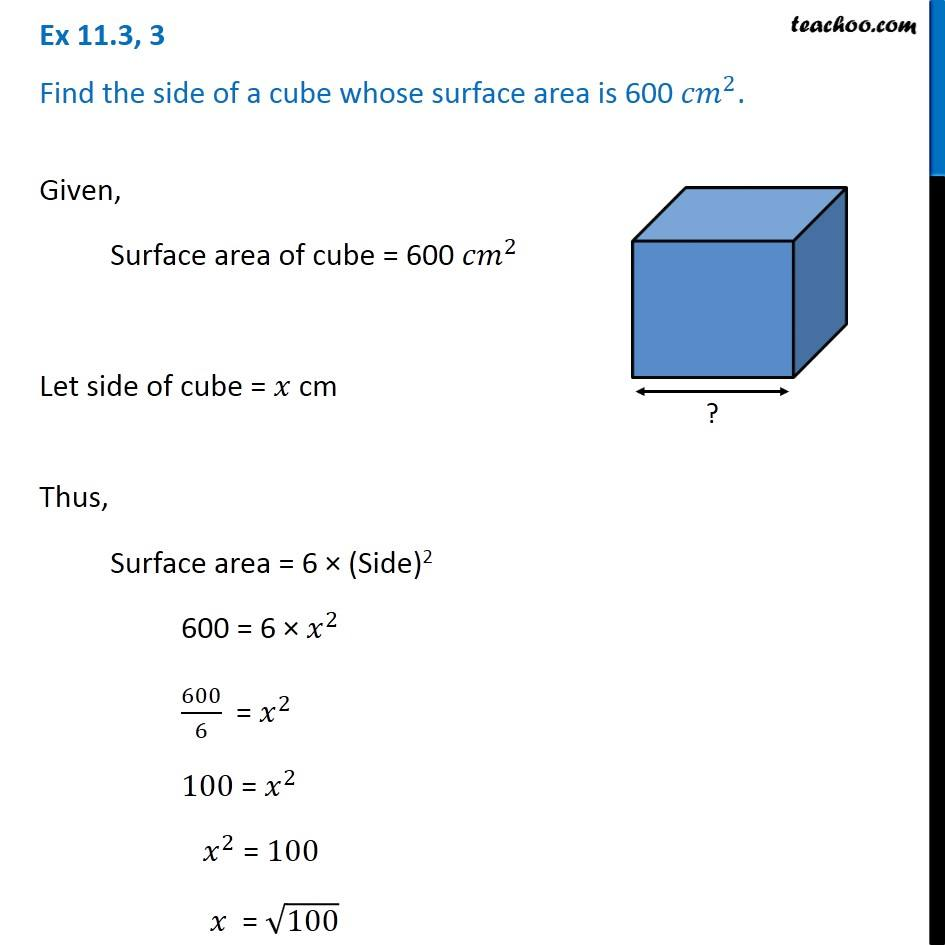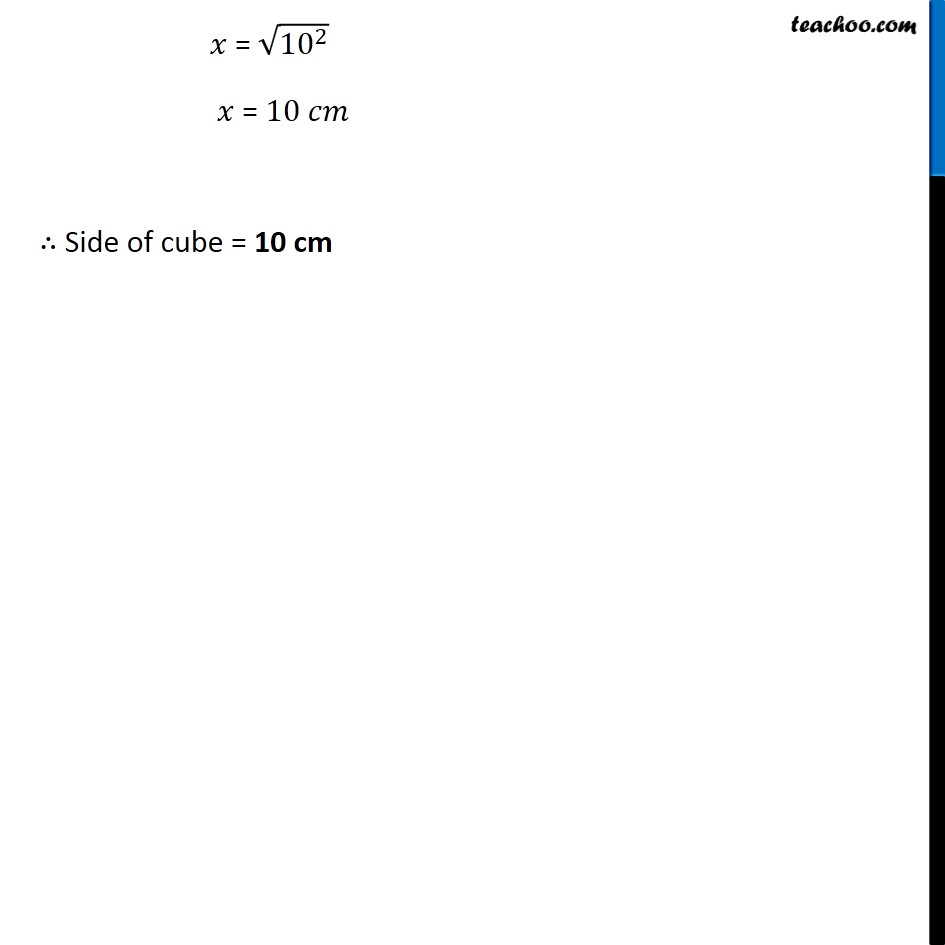Chapter 9 Class 8 Mensuration
Concept wiseLearn in your speed, with individual attention - Teachoo Maths 1-on-1 Class

### Transcript

Ex 9.2, 3 Find the side of a cube whose surface area is 600 〖𝑐𝑚〗^2. Given, Surface area of cube = 600 〖𝑐𝑚〗^2 Let side of cube = 𝑥 cm Thus, Surface area = 6 × (Side)2 600 = 6 × 𝑥^2 600/6 = 𝑥^2 100 = 𝑥^2 𝑥^2 = 100 𝑥 = √100 𝑥 = √(〖10〗^2 ) 𝑥 = 10 𝑐𝑚 ∴ Side of cube = 10 cm